Next: Advantage of the Hamiltonian Up: Laser-driven particle mechanics Previous: Motivational Overview   Contents

# Lagrangian and Hamiltonian Formulation of Mechanics.

The action integral for a particle of mass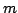and charge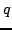is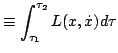(1)

where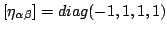andis the given electromagnetic vector potential. The integral I is an extremum for those worldlines between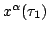and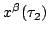which satisfy the Euler-Lagrange equationnamely,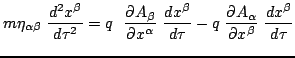orwith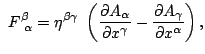which are the Lorentz equations of motion for the charged particle.

A physically and mathematically more advantageous set of equations is based on the introduction of the momentum variables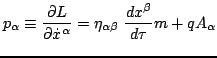and the superhamiltonian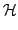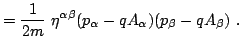(2)

In terms of these one has the two sets of Hamiltonian equations of motion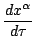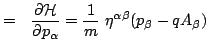(3) and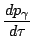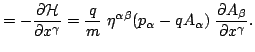(4)

One can readily check that this system of first order equations implies the Euler-Lagrange equations.Next: Advantage of the Hamiltonian Up: Laser-driven particle mechanics Previous: Motivational Overview   Contents
Ulrich Gerlach 2005-11-07